Next: Equilibrium of a laminar Up: Statics Previous: Introduction

## The principles of statics

Consider a general extended body which is subject to a number of external forces. Let us model this body as a swarm ofpoint particles. In the limit that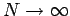, this model becomes a fully accurate representation of the body's dynamics.

In Sect. 6.3 we determined that the overall translational equation of motion of a general-component system can be written in the form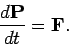(456)

Here,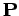is the total linear momentum of the system, and(457)

is the resultant of all the external forces acting on the system. Note that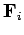is the external force acting on the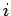th component of the system.

Equation (456) effectively determines the translational motion of the system's centre of mass. Note, however, that in order to fully determine the motion of the system we must also follow its rotational motion about its centre of mass (or any other convenient reference point). In Sect. 9.4 we determined that the overall rotational equation of motion of a general-component system (with central internal forces) can be written in the form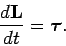(458)

Here,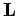is the total angular momentum of the system (about the origin of our coordinate scheme), and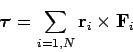(459)

is the resultant of all the external torques acting on the system (about the origin of our coordinate scheme). In the above,is the vector displacement of theth component of the system.

What conditions must be satisfied by the various external forces and torques acting on the system if it is to remain stationary in time? Well, if the system does not evolve in time then its net linear momentum,, and its net angular momentum,, must both remain constant. In other words,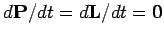. It follows from Eqs. (456) and (458) that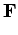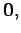(460)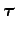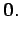(461)

In other words, the net external force acting on system must be zero, and the net external torque acting on the system must be zero. To be more exact:
The components of the net external force acting along any three independent directions must all be zero;
and
The magnitudes of the net external torques acting about any three independent axes (passing through the origin of the coordinate system) must all be zero.
In a nutshell, these are the principles of statics.

It is clear that the above principles are necessary conditions for a general physical system not to evolve in time. But, are they also sufficient conditions? In other words, is it necessarily true that a general system which satisfies these conditions does not exhibit any time variation? The answer to this question is as follows: if the system under investigation is a rigid body, such that the motion of any component of the body necessarily implies the motion of the whole body, then the above principles are necessary and sufficient conditions for the existence of an equilibrium state. On the other hand, if the system is not a rigid body, so that some components of the body can move independently of others, then the above conditions only guarantee that the system remains static in an average sense.

Before we attempt to apply the principles of statics, there are a couple of important points which need clarification. Firstly, does it matter about which point we calculate the net torque acting on the system? To be more exact, if we determine that the net torque acting about a given point is zero does this necessarily imply that the net torque acting about any other point is also zero? Well,(462)

is the net torque acting on the system about the origin of our coordinate scheme. The net torque about some general pointis simply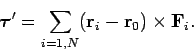(463)

However, we can rewrite the above expression as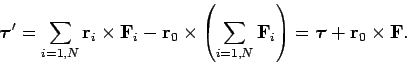(464)

Now, if the system is in equilibrium then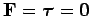. Hence, it follows from the above equation that(465)

In other words, for a system in equilibrium, the determination that the net torque acting about a given point is zero necessarily implies that the net torque acting about any other point is also zero. Hence, we can choose the point about which we calculate the net torque at will--this choice is usually made so as to simplify the calculation.

Another question which needs clarification is as follows. At which point should we assume that the weight of the system acts in order to calculate the contribution of the weight to the net torque acting about a given point? Actually, in Sect. 8.11, we effectively answered this question by assuming that the weight acts at the centre of mass of the system. Let us now justify this assumption. The external force acting on theth component of the system due to its weight is(466)

where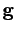is the acceleration due to gravity (which is assumed to be uniform throughout the system). Hence, the net gravitational torque acting on the system about the origin of our coordinate scheme is(467)

where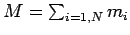is the total mass of the system, and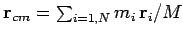is the position vector of its centre of mass. It follows, from the above equation, that the net gravitational torque acting on the system about a given point can be calculated by assuming that the total mass of the system is concentrated at its centre of mass.Next: Equilibrium of a laminar Up: Statics Previous: Introduction
Richard Fitzpatrick 2006-02-02